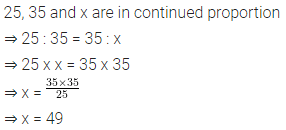# Selina Concise Mathematics Class 6 ICSE Solutions Chapter 12 Proportion

## Selina Concise Mathematics Class 6 ICSE Solutions Chapter 12 Proportion (Including Word Problems)

Selina Publishers Concise Mathematics Class 6 ICSE Solutions Chapter 12 Proportion (Including Word Problems)

### Proportion Exercise 12A – Selina Concise Mathematics Class 6 ICSE Solutions

Question 1.
In each of the following, check whether or not the given ratios form a proportion :
(i) 8 : 16 and 12 : 15
(ii) 16 : 28 and 24 : 42
(iii) 12 ÷ 3 and 8 ÷ 2
(iv) 25 : 40 and 20 : 32
(v) $$\frac { 15 }{ 18 } and \frac { 10 }{ 12 }$$
(vi) $$\frac { 7 }{ 8 }$$ and 14 : 16
Solution: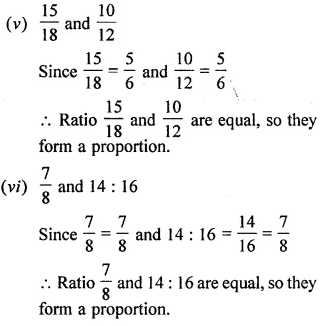Question 2.
Find the value of x in .each of the following proportions :
(i) x : 4 = 6 : 8
(ii) 14 : x = 7 : 9
(iii) 4 : 6 = x : 18
(iv) 8 : 10 = x : 25
(v) 5 : 15 = 4 : x
(vi) 16 : 24 = 6 : x
Solution: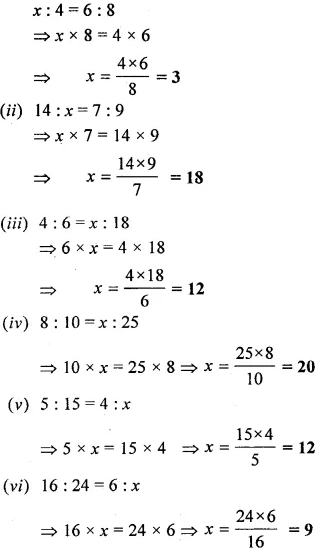Question 3.
Find the value of x so that the given four numbers are in proportion :
(i) x, 6, 10 and 15
(ii) x, 4, 15 and 30
(iii) 2, x, 10 and 25
(iv) 4, x, 6 and 18
(v) 9, 12, x and 8
(vi) 4, 10, 36 and x
(vii) 7, 21, x and 45
(viii) 6, 8, 12 and x.
Solution: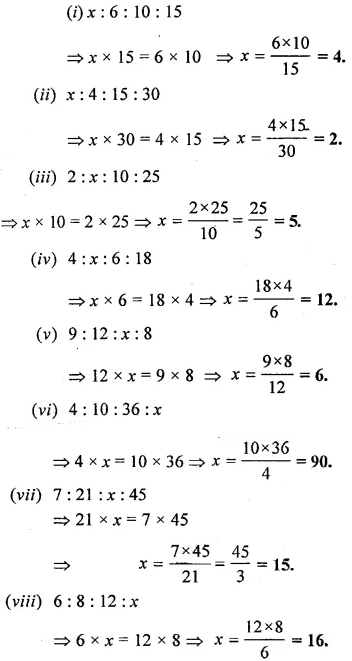Question 4.
The first, second and the fourth terms of a proportion are 6, 18 and 75, respectively. Find its third term.
Solution: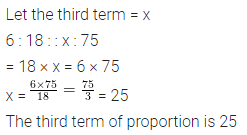Question 5.
Find the second term of the proportion whose first, third and fourth terms are 9, 8 and 24 respectively.
Solution:Question 6.
Find the fourth term of the proportion whose first, second and third terms are 18, 27, and 32 respectively.
Solution:Question 7.
The ratio of the length and the width of a school ground is 5 : 2. Find the length, if the width is 40 metres.
Solution: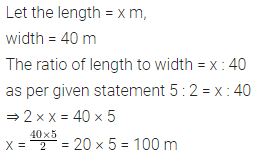Question 8.
The ratio of the sale of eggs on a Sunday and that of the whole week at a grocery shop was 2 : 9. If the total value of the sale of eggs in the same week was Rs 360, find the value of the sale of eggs that Sunday.
Solution: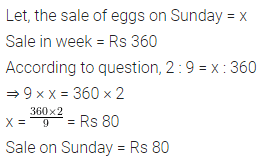Question 9.
The ratio of copper and zinc in an alloy is 9 : 8. If the weight of zinc, in the alloy, is 9.6 kg ; find the weight of copper in the alloy.
Solution:Question 10.
The ratio of the number of girls to the number of boys in a school is 2 : 5. If the number of boys is 225 ; find:
(i) the number of girls in the school.
(ii) the number of students in the school.
Solution: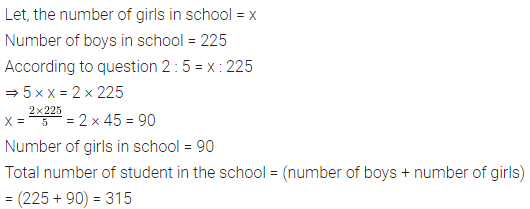Question 11.
In a class, one out of every 5 students pass. If there are 225 students in all the sections of a class, find how many pass ?
Solution:Question 12.
Make set of all possible proportions from the numbers 15, 18, 35 and 42.
Solution:### Proportion Exercise 12B – Selina Concise Mathematics Class 6 ICSE Solutions

Question 1.
If x, y and z are in continued proportion, then which of the following is true :
(i) x : y = x : z
(ii) x : x = z : y
(iii) x : y = y : z
(iv) y : x = y : z
Solution: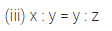Question 2.
Which of the following numbers are in continued proportion :
(i) 3, 6 and 15
(ii) 15, 45 and 48
(iii) 6, 12 and 24
(iv) 12, 18 and 27
Solution:Question 3.
Find the mean proportion between
(i) 3 and 27
(ii) 0.06 and 0.96
Solution: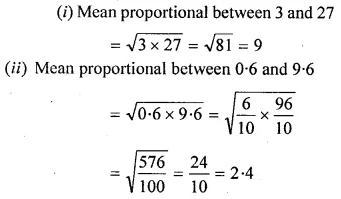Question 4.
Find the third proportional to :
(i) 36, 18
(ii) 5.25, 7
(iii) ₹ 1.60, ₹ 0.40
Solution: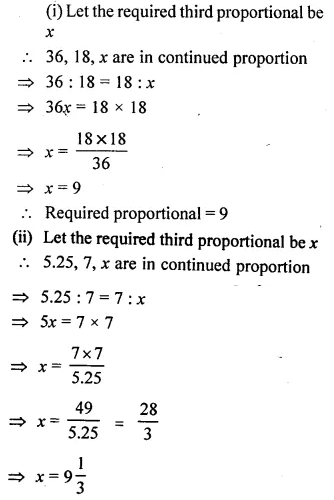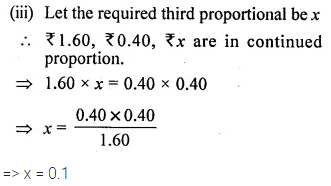Question 5.
The ratio between 7 and 5 is same as the ratio between ₹ x and ₹ 20.50 ; find the value of x.
Solution: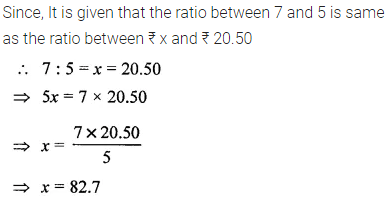Question 6.
If (4x + 3y) : (3x + 5y) = 6 : 7, find :
(i) x : y
(ii) x, if y = 10
(iii) y, if x = 27
Solution: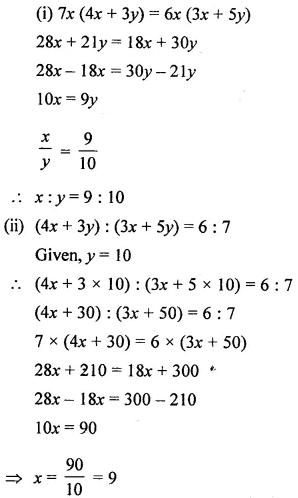Question 7.
If $$\frac { 2y+5x }{ 3y-5x } =2\frac { 1 }{ 2 }$$, find:
(i) x : y
(ii) x, if y = 70
(iii) y, if x = 33
Solution: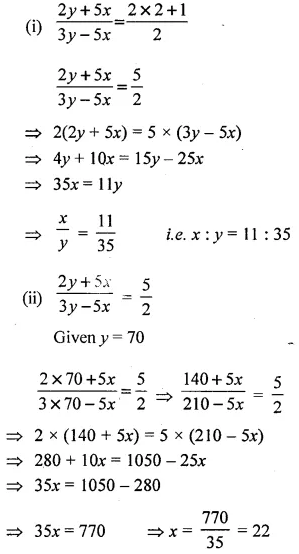### Proportion Exercise 12C – Selina Concise Mathematics Class 6 ICSE Solutions

Question 1.
Are the following numbers in proportion:
(i) 32, 40, 48 and 60 ?
(ii) 12,15,18 and 20 ?
Solution:Question 2.
Find the value of x in each of the following such that the given numbers are in proportion.
(i) 14, 42, x and 75
(ii) 45, 135, 90 and x
Solution:Question 3.
The costs of two articles are in the ratio 7 : 4. If the cost of the first article is Rs. 2,800 ; find the cost of the second article.
Solution: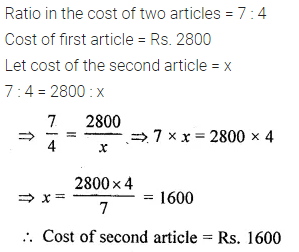Question 4.
The ratio of the length and the width of a rectangular sheet of paper is 8 : 5. If the width of the sheet is 17.5 cm; find the length.
Solution:Question 5.
The ages of A and B are in the ratio 6 : 5. If A’s age is 18 years, find the age of B.
Solution: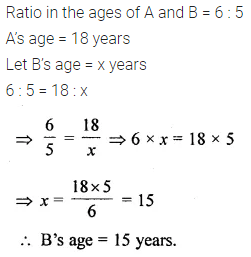Question 6.
A sum of Rs. 10, 500 is divided among A, B and C in the ratio 5 : 6 : 4. Find the share of each.
Solution: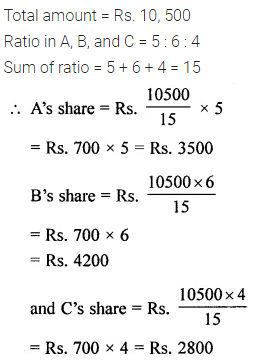Question 7.
Do the ratios 15 cm to 2 m and 10 sec to 3 minutes form a proportion ?
Solution:Question 8.
Do the ratios 2 kg : 80 kg and 25 g : 625 g form a proportion ?
Solution:Question 9.
10 kg sugar cost ₹ 350. If x kg sugar of the same kind costs ₹ 175, find the value of x
Solution:Question 10.
The length of two ropes are in the ratio 7 : 5. Find the length of:
(i) shorter rope, if the longer one is 22.5 ni
(ii) longer rope, if the shorter is 9.8 m.
Solution: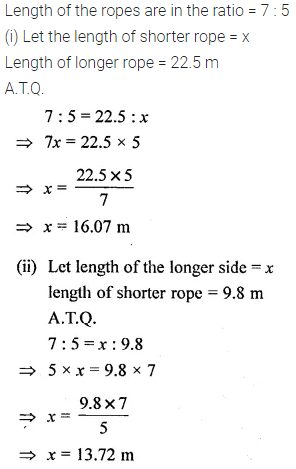Question 11.
If 4, x and 9 are in continued proportion, find the value of x.
Solution: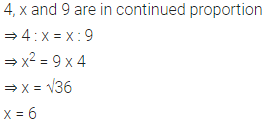Question 12.
If 25, 35 and x are in continued proportion, find the value of x.
Solution: